## MATLAB codes used in "Classifying Matrices with a Spectral Regularization" (ICML 2007)

### What it does

It solves a classification problem over symmetric matrices with dual spectral norm (trace norm) regularization using a simple interior point method. It was successfully applied to single trial EEG classification problem in the context of brain-computer interfacing (BCI). In this case the input matrix is the short-time covariance estimate or its matrix logarithm (Tomioka & Aihara, 2007; see also [Slides]).

Given training examples (X1,y1), (X2,y2),..., (Xn,yn), it solves a regularized empirical risk minimization problem: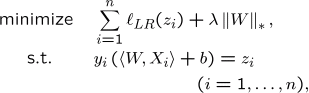where W is a real matrix and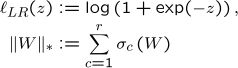The above norm (sum of singularvalues) is called the dual spectral norm, trace norm, Ky-Fan r-norm, or the nuclear norm.

### Results

BCI competition III dataset IVa.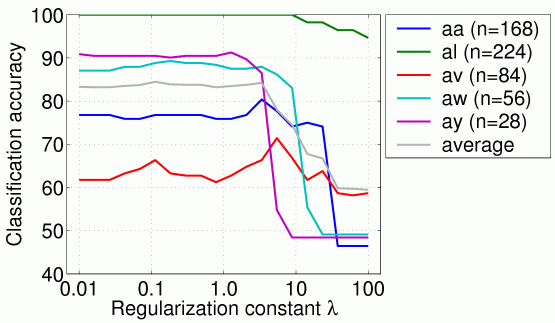(a) inputs are covariance matrices (symmetric and positive semidefinite)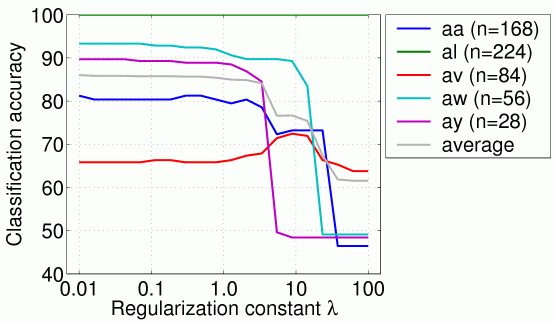(b) inputs are the log of covariance matrices (only symmetric)

You can compare the above results with the results at the competition. Note that the task in the competition was to cope with the small training set size and many participants used the test data to adaptly update their classifiers, which we haven't done here for the sake of simplicity.

### How to use it

2. Download the datasets: BCI competition III dataset IVa. Download also the true labels, save it with the variable name true_y.
3. Change the first two lines of BcicompIIIiva.m to the path you saved the datasets, as follows:
4. 4:       file   = '<your path>data_set_IVa_%s.mat';

(%s is where the name of each subject is substituted.)
5. Run the script BcicompIIIiva.m.
6. You can compare the results with the files result.mat and performance_acc.eps in the folder results.
7. You can try the logm option by setting opt.logm to one as follows:
8. 13:      opt.logm = 1;

The results are saved in files result_logm.mat and performance_logm_acc.eps.
9. You can also try another implementation based on CVX toolbox, developed by Michael Grant, Stephen Boyd, and Yinyu Ye (tested with version 1.1 build 520 July 29, 2007). Call lrds_cvx.m instead of lrds_dual.m as follows:
63:      [W, bias] = lrds_cvx(Xtr, Ytr, lambda(ii));

Note that the number of electrodes is reduced to 49 (see channels.eps) to make the computation fast. You can skip this but we have seen no significant difference in the performance.

### List of files

Here's a list of files created using m2html. You can also take a look into the files.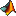BcicompIIIiva BcicompIIIiva.m - main script file that applies the method to BCIapply_lrds apply_lrds - applies the classifiercovariance covariance - calculate the covariance between channels for each samplecutoutTrials cutoutTrials - cuts out trials from continuous EEG recordinggetfieldarray getfieldarray - get field from a structured array.logmatrix logmatrix - takes logm for each trial.loss_0_1 loss_0_1 - zero-one loss.lrds_cvx lrds_cvx - logistic regression with dual spectral regularizationlrds_dual lrds_dual - Logistic regression with dual spectral regularizationmatmultcv matmultcv - multiplies matrix to each covarianced trial.setDefaults setDefaults - set defaults.train_lrds train_lrds - wrapper function that produces the classifier structwhiten whiten - Perform whitening

Generated on Sat 26-Apr-2008 15:48:22 by m2html © 2003

### Request

• Please cite the following paper if you publish something based on the software provided above:
• "Classifying Matrices with a Spectral Regularization",
Ryota Tomioka and Kazuyuki Aihara, Proc. of the 24th Annual International Conference on Machine Learning (ICML2007), pp. 895-902, ACM Press. Oregon, USA, June, 2007. [BibTex]

• Please keep the copyright information in the files and provide a link to this page if you make a software based on the codes provided above public.

### Related studies

I give the list of relevant prior studies below because I was not aware of them when my ICML paper was written.

### Acknowledgments

I am grateful to Klaus-Robert Müller and my colleagues at Intelligent Data Analysis Group, Fraunhofer FIRST and Technische Universität Berlin for their support in developing this software. I thank Jeremy Hill at Max Planck Institiute for Biological Cybernetics for suggesting me the logm variant.

### Contact

Ryota Tomiokaback to my homepage